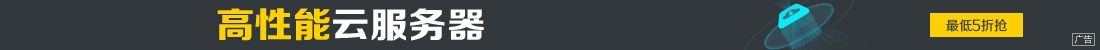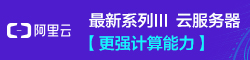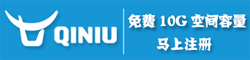# 深入理解js中this的用法

••this是js的一个关键字，随着函数使用场合不同，this的值会发生变化。但是总有一个原则，那就是this指的是调用函数的那个对象。

1、纯粹函数调用。

```function test() {
this.x = 1;
}

test();```

```var x = 1;
function test() {
}
test();//1

var x = 1;
function test() {
this.x = 0;
}
test();

2、作为方法调用，那么this就是指这个上级对象。

```function test() {
}

var o = {};
o.x = 1;
o.m = test;
o.m(); //1
```

3、作为构造函数调用。所谓构造函数，就是生成一个新的对象。这时，这个this就是指这个对象。

```function test() {
this.x = 1;
}
var o = new test();

4、apply调用

```var x = 0;
function test() {
}

var o = {};
o.x = 1;
o.m = test;
o.m.apply(); //0
o.m.apply(o);//1```